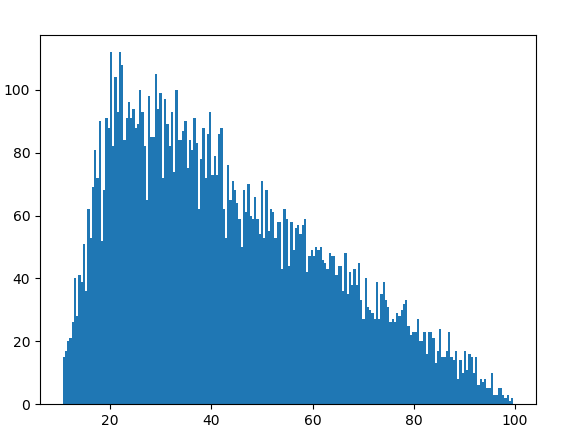# random.triangular() method in Python

`triangular()` is an inbuilt method of the `random` module. It is used to return a random floating point number within a range with a bias towards one extreme.

Syntax : random.triangular(low, high, mode)

Parameters :
low : the lower limit of the random number
high : the upper limit of the random number
mode : additional bias; low < mode < high

if the parameters are (10, 100, 20) then due to the bias, most of the random numbers generated will be closer to 10 as opposed to 100.

Returns : a random floating number

Example 1:

 `# import the random module ` `import` `random ` ` `  `# determining the values of the parameters ` `low ``=` `10` `high ``=` `100` `mode ``=` `20` ` `  `# using the triangular() method ` `print``(random.triangular(low, high, mode)) `

Output :

`22.614510550239572`

Example 2: If we generate the number multiple times we can probably identify the bias.

 `# import the random module ` `import` `random ` ` `  `# determining the values of the parameters ` `low ``=` `10` `high ``=` `100` `mode ``=` `20` ` `  `# running the triangular method with the ` `# same parameters multiple times ` `for` `i ``in` `range``(``10``): ` `    ``print``(random.triangular(low, high, mode)) `

Output :

```58.645768016894735
46.690692250503226
33.57590419190895
52.331804090351305
33.09451214875767
12.03845752596168
32.816080679206294
20.4739124559502
82.49208123077557
63.511062284733015```

Example 3: We can visualize the triangular pattern by plotting a graph.

 `# import the required libraries ` `import` `random ` `import` `matplotlib.pyplot as plt ` ` `  ` `  `# store the random numbers in a list ` `nums ``=` `[] ` `low ``=` `10` `high ``=` `100` `mode ``=` `20` ` `  `for` `i ``in` `range``(``10000``): ` `    ``temp ``=` `random.triangular(low, high, mode) ` `    ``nums.append(temp) ` `     `  `# plotting a graph ` `plt.hist(nums, bins ``=` `200``) ` `plt.show() `

Output :Whether you're preparing for your first job interview or aiming to upskill in this ever-evolving tech landscape, GeeksforGeeks Courses are your key to success. We provide top-quality content at affordable prices, all geared towards accelerating your growth in a time-bound manner. Join the millions we've already empowered, and we're here to do the same for you. Don't miss out - check it out now!

Previous
Next